#### 期刊菜单

Design and Implementation of Wireless Communication System Based on Digital Coding Metasurface
DOI: 10.12677/HJWC.2019.92008, PDF, HTML, XML, 下载: 1,009  浏览: 3,451

Abstract: Artificial electromagnetic metamaterials continue to receive the attention of researchers due to their unique physical properties. There are many application examples of metamaterials in various fields such as digital imaging, electromagnetics, and radar. This paper designs and implements a wireless communication system using a digital coding metasurface (one kind of the metamaterials) to establish a new bridge between electromagnetic metamaterials and wireless communication. The system utilizes a digital coding metasurface to perform direct modulation of the wireless signal from the baseband to the radio frequency. In addition, the experiment based on LabVIEW FPGA proves that the wireless communication system based on metasurface can transmit pictures smoothly and stably at 3.8 GHz with FSK modulation. This combination of metasurface and wireless communication also provides a new idea and possibility to build a new communication system architecture.

1. 引言

2. 数字可编程超表面无线通信系统模型

2.1. 数字可编码超表面的结构及载波调制原理Figure 1. Structure diagram of digital coding metasurface

${R}_{n}\left(t\right)={\Gamma }_{n}\left(t\right)\cdot {I}_{n}\left(t\right)$ (1)

2.2. 不同数字调制方案下发射机对超表面数字驱动电压序列的设计

$\Gamma \left(t\right)={\Gamma }_{m}h\left(t\right),0\le t\le T,{\Gamma }_{m}\in A$ (2)

${\Gamma }_{m}\in A=\left\{{e}^{j\frac{\text{π}}{4}},{e}^{j\frac{3\text{π}}{4}},{e}^{j\frac{5\text{π}}{4}},{e}^{j\frac{7\text{π}}{4}}\right\},|A|=4,m=0,1,2,3$ (3)

$\Gamma \left(t\right)={\Gamma }_{m}h\left(t\right),0\le t\le T$ (4)

${\Gamma }_{m}={e}^{j2\text{π}{f}_{m}t},{f}_{m}\in A=\left\{{f}_{0},\cdots ,{f}_{M-1}\right\}$ (5)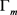Figure 2. The modulation processes of the transmitter in a metasurface-based single-carrier wireless communication system

$\Gamma \left(t\right)={\sum }_{p}^{P-1}{\Gamma }_{p}g\left(t-p\tau \right),0\le t\le T,{\Gamma }_{p}\in A$ (6)

$g\left(t\right)=1,0\le t\le \tau$ (7)

$\Gamma \left(t\right)={\sum }_{k=-\infty }^{+\infty }{a}_{k}{e}^{jk2\text{π}{f}_{0}t},0\le t\le T$ (8)

$g\left(t\right)={\sum }_{k=-\infty }^{+\infty }{c}_{k}{e}^{jk2\text{π}{f}_{0}t},0\le t\le \tau$ (9)

${c}_{k}=\frac{1}{P}Sa\left(\frac{k\pi }{P}\right){e}^{-j\frac{k\text{π}}{P}}$ (10)

$\begin{array}{l}\Gamma \left(t\right)={\sum }_{p=0}^{P-1}{\Gamma }_{p}g\left(t-p\tau \right)\\ \text{=}{\sum }_{k=-\infty }^{+\infty }{c}_{k}\left({\sum }_{p=0}^{P-1}{\Gamma }_{p}{g}^{-j\frac{2kp\text{π}}{P}}\right){e}^{jk2\text{π}{f}_{0}t},0\le t\le T\end{array}$ (11)

${a}_{k}={A}_{k}{\sum }_{p=0}^{P-1}{\Gamma }_{p}{e}^{-j\frac{2kp\text{π}}{P}}$ (12)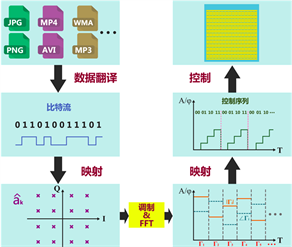Figure 3. The modulation processes of the transmitter in a metasurface-based multi-carrier wireless communication system

2.3. 未来超表面无线通信系统可能的结构和应用方式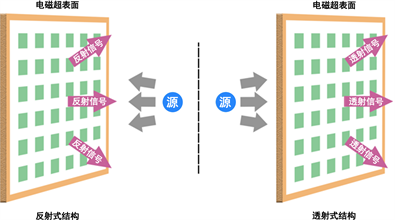Figure 4. Reflective and transmissive structures of metasurface

3. 数字可编程超表面无线通信系统的硬件实现

3.1. 系统架构和参数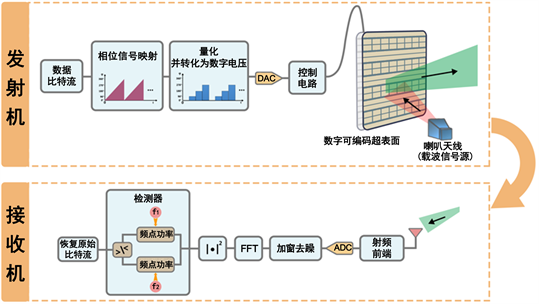Figure 5. Diagram of metasurface-based FSK wireless communication systemTable 1. List of devices and hardwareTable 2. List of system parameters

3.2. 收发端关键代码及操作

LabVIEWFPGA是美国国家仪器公司推出的一款图形化界面FPGA开发语言，具有易开发、可释性强等优点，本文采用该语言进行数字可编程超表面无线通信系统的实现。下面给出该系统收发端关键的关键操作和模块代码。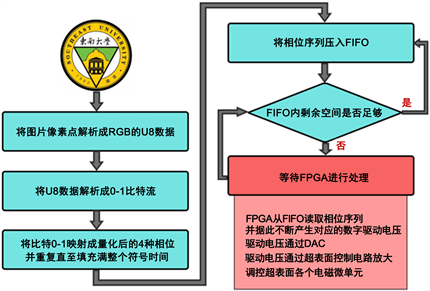Figure 6. Flowchart of converting bit stream into sequences of phase and digital driving voltages in transmitter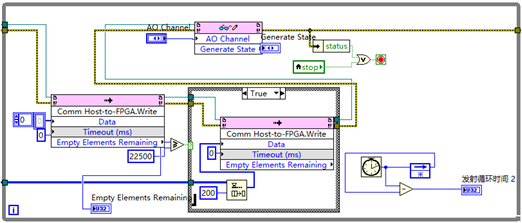Figure 7. Feeding FPGA with sequences of phase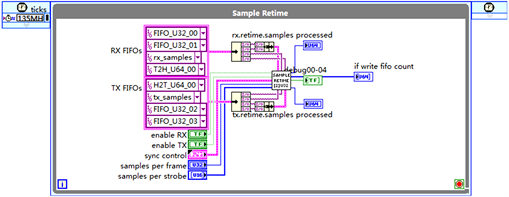Figure 8. Loop of sampling retiming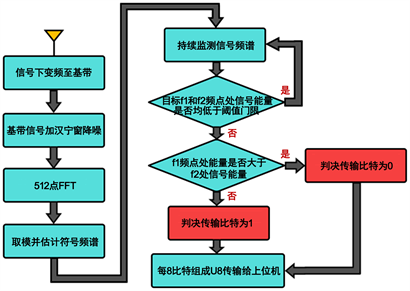Figure 9. Flowchart of baseband processing in receiver

3.3. 测试结果及讨论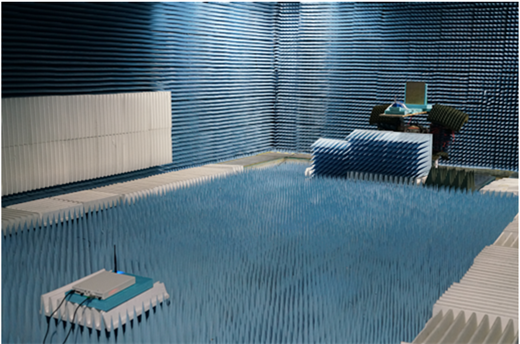Figure 10. The experimental metasurface-based FSK wireless communication system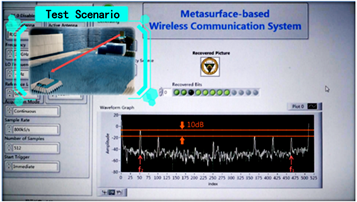Figure 11. The experiment results of metasurface-based FSK wireless communication system

4. 结论

  Holloway, C.L., Kuester, E.F., Gordon, J.A., OHara, J., Booth, J. and Smith, D.R. (2012) An Overview of the Theory and Applica-tions of Metasurfaces: The Two-Dimensional Equivalents of Metamaterials. IEEE Antennas and Propagation Magazine, 54, 10-35. https://doi.org/10.1109/MAP.2012.6230714  Pereda, A.T., Caminita, F., Martini, E. and Ederra, I. (2018) Experimental Validation of a Ku-Band Dual-Circularly Polarized Metasurface Antenna. IEEE Transactions on Antennas and Propagation, 66, 1153-1159. https://doi.org/10.1109/TAP.2018.2794395  Zvolensky, T., Gollub, J.N., Marks, D.L. and Smith, D.R. (2017) Design and Analysis of a W-Band Metasurface-Based Computational Imaging System. IEEE Access, 5, 9911-9918. https://doi.org/10.1109/ACCESS.2017.2703860  Cui, T.J., Liu, S. and Li, L.L. (2016) Information Entropy of Coding Metasurface. Light: Science & Applications, 5, e16172. https://doi.org/10.1038/lsa.2016.172  纪静文, 蔡超时, 胡峰, 梁云英. OFDM系统中CF-ACE PAPR技术对功放非线性的抑制[J]. 中国传媒大学学报自然科学版, 2011, 18(3): 23-29.  力智, 胡学龙. 单载波与多载波调制系统中调制识别算法研究[J]. 国外电子测量技术, 2016, 35(11): 73-75.  周乐柱, 李斗, 郭文嘉. 卫星通信多波束天线综述[J]. 电子学报, 2001, 29(6): 824-828.  吴迪, 田茂, 皮楚, 何昉明. 共形天线阵列极化分集问题[J]. 太赫兹科学与电子信息学报, 2018, 16(3): 445-451.  Liaskos, C. and Nie, S. (2018) A New Wireless Communication Paradigm through Software-Controlled Metasurfaces. IEEE Communications Magazine, 56, 162-169. https://doi.org/10.1109/MCOM.2018.1700659  Huang, C.H. and Alexandropoulos, G.C. (2018) Energy Efficient Mul-ti-User MISO Communication Using Low Resolution Large Intelligent Surfaces. IEEE GLOBECOM. arXiv:1809.05397.